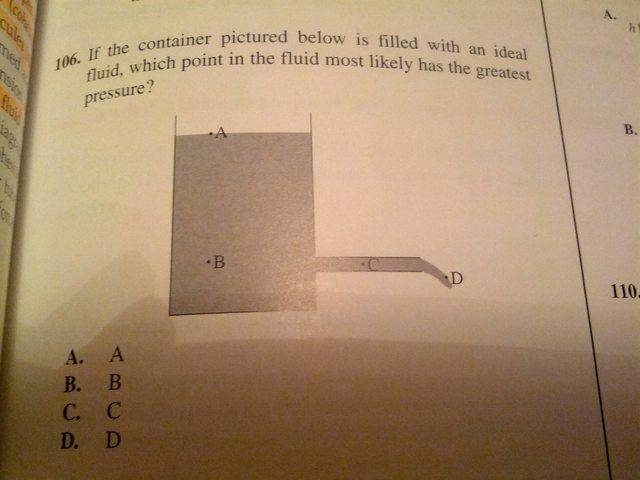Fluid Pressure

Homework StatementHomework Equations

fluid pressure = fluid density * depth * g

The Attempt at a Solution

My understanding is that fluid pressure at any two points at the same depth is equal, for a fluid at rest. But because one end is open, the pressure at point C is less than B and equal to A? I don't understand intuitively how this works.

Any help would be appreciated - thanks!

Simon Bridge
Homework Helper
Well at point A - what is exerting the pressure?

Would this be a high or a low pressure compared with the rest?

Remains to consider B and C ... what is exerting the pressure in each case?
Is there anything else that would contribute to the pressure?

At point A, we have atmospheric pressure, as does point D, so they are equal I think. B has the weight of the water molecules above it, so it experiences more pressure than A and D. I'm just not sure why C is < B when they are at the same depth. Is it because molecules at C are moving but not at B?

Simon Bridge